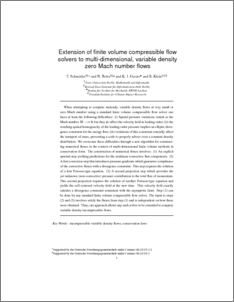Repository: Freie Universität Berlin, Math Department

# Extension of finite volume compressible flow solvers to multidimensional, variable density zero Mach number flows

Schneider, T. and Botta, N. and Geratz, K. J. and Klein, R. (1999) Extension of finite volume compressible flow solvers to multidimensional, variable density zero Mach number flows. J. Comput. Phys., 155 . pp. 248-286.Preview

2MB

## Abstract

When attempting to compute unsteady, variable density flows at very small or zero Mach number using a standard finite volume compressible flow solver one faces at least the following difficulties: (i) Spatial pressure variations vanish as the Mach numberM

Item Type: Article Mathematical and Computer Sciences > Mathematics > Applied Mathematics Department of Mathematics and Computer Science > Institute of Mathematics > Geophysical Fluid Dynamics Group 479 Ulrike Eickers 26 Jun 2009 08:33 03 Mar 2017 14:40

Repository Staff Only: item control page